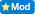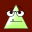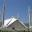how can i simplify 3x/2 - 4x/5 ?

0  Views: 548 Answers: 3 Posted: 8 years ago
ColleenBelow comment move to the comments section as it does not answer the question.
From the rules page: "If the answer is not helpful or informative but is posted as a new answer, it will be moved to the appropriate area." http://www.akaqa.com/rules
________________________________________________________________An Old Friend
Karma: 3495

If you are not capable of doing so, ask your teacher for assistance, WBMA

Colleen__________________________________________________________LOG IN
Karma: 50435

Don't you think it would be easier after taking L.C.M .

3x/2 - 4×/5 =15×/10 - 8×/10 = 7×/10

10 is the lowest common multiple, but you do not substract denominators. If you did, the answer would always be zero.

3x            4x

____   -    ____

2              5

Find LCD > 10

15x               8x

_____   -    _______

10                10

____

10

3x/2-4x/5

STEP 1    find the LCF between denominators which is 2 & 5

and is 10 since 2 is able to go into 10 5times whiles 5 is also able to go into 10 2times and equat it to 0(zero)

STEP 2     change it from fraction into linear form by by multiply 3x/2 by 10 vice versa

STEP3      subtract 8x from 15x which is 7x =0

STEP4      divide through by 7 to find x and x is zero.

3x/2-4x/5=0

10*3x/2-10*4x/5=0

15x-8x=o

7x=0

7x/7=0/7

x=0

### Top contributors in Homework Help category

ROMOS
Karma: 15225

country bumpkin
Karma: 10395

Benthere
Karma: 8625

Colleen